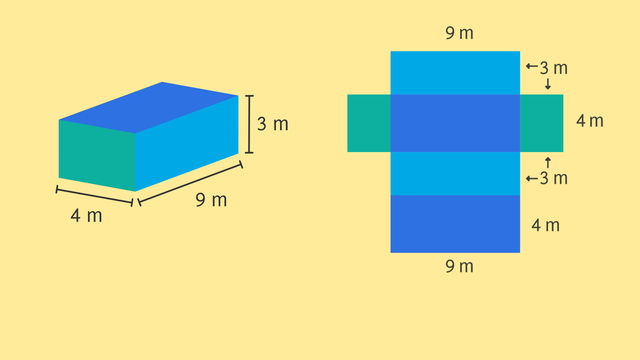# Using Nets to Find Surface AreaRating

Ø 3.5 / 2 ratings
The authorsChris S.

## Basics on the topicUsing Nets to Find Surface Area

After this lesson, you will be able to use a net to determine the surface area of a rectangular prism and a square pyramid.

The lesson begins by teaching you that a net is a 2 dimensional representation of a 3 dimensional figure. It leads you to learn that the surface area of a rectangular prism is the sum of the areas of pairs of rectangles. It concludes with the surface area of a square pyramid, which is the sum of the areas of a square and four identical triangles.

Learn about nets by helping Dwayne and Steve play pranks on each other!

This video includes key concepts, notation, and vocabulary such as a net (a 2 dimensional representation of a 3 dimensional figure) and surface area (the sum of the areas of the faces of a 3 dimensional figure).

Before watching this video, you should already be familiar with prisms and pyramids, and how to calculate area of rectangles and triangles.

After watching this video, you will be prepared to learn to find surface area of more complex 3 dimensional figures.

Common Core Standard(s) in focus: 6.G.A.2 and 6.GA.4 A video intended for math students in the 6th grade Recommended for students who are 11 - 12 years old

### TranscriptUsing Nets to Find Surface Area

After months of persistence, Dwayne has earned the prestigious title of Assistant Regional Resident Assistant! He's quite proud of his title and that means his arch-nemesis Steve HAS to play a prank on him. Let's see what Steve's got up his sleeve! Steve's gonna cover Dwayne's room with sticky notes! How many notes will it take? We can use nets to find the surface area of the room to find out! The room is a rectangular prism that is 4 meters wide 3 meters tall and 9 meters long. We can unfold this rectangular prism to get a net, or 2 dimensional flattening of the prism. A net is useful because, with a net, we can easily see each face, or two-dimensional side, of the prism. There are 6 faces. Because opposite faces of the prism are the same there are 3 pairs of identical faces here, here and here. The aurface area of the prism is the SUM of the areas of all the faces. Each face is a rectangle. And we know that the area of each rectangle is its length times its width. So to find the surfface area of this rectangular prism, we need to find the area of each pair of rectangles making up the prism, and then add all of these areas together to get the surface area of Dwayne's room. Let's start with these two. The area of each is 3 times 4, which is 12 square meters. Now let's look at these two. The area is 3 times 9, which is 27 square meters. Now, these two. 4 times 9 is 36. To calculate the surface area, we can now plug in 12 for the area of face one and the face 2, 27 for area of face three and face 4 and 36 for the area of face five and face six. Simplifying, we get 2 times 12 plus 2 times 27 plus 2 times 36. Simplifying further, we have 24 plus 54 plus 72 for a total surface area of 150 square meters. Now that Steve knows the surface area of Dwayne's room, he can get to work on posting the post-its! Steve's gotta work fast before Dwayne returns! Where IS Dwayne anyway? Oh man, Dwayne is up to shenanigans of his own in Steve's room! What's this? A blueprint for something devious it seems. We've got a square base that's 3 meters by 3 meters and four triangular lateral faces that have a height of 4.5 meters. This looks like a net for a 3-dimensional shape but what shape is it? That's right! It's the net of a square pyramid. Let's find the surface area of this square pyramid by using its net to visualize its faces. The area of the square is 3 times 3 which is 9 square meters. The area of each triangle is half the base length, times the height. That's one-half, times 3, times 4.5. That's half of 13.5, or 6.75 square meters. The surface area of this square pyramid is the sum of the areas of its faces. There are four triangles so the total surface area is 9, plus four times 6.75. That's 9 plus 27, or 36 square meters. Now Dwayne is ready to construct his pyramid scheme. Before we see which guy gets caught net-handed, let's review how we can use nets to find surface area. We can unfold rectangular prisms and square pyramids to get a 2 dimensional version called a net. We can then use a net to find the surface area by calculating the area of each face and then adding those areas together. Oh man, they finished at the same time!

1 comment
1 comment
1.I love this! 3D is difficult to visualise, makes it very easy to understand

From Suziegardiner9, over 3 years ago